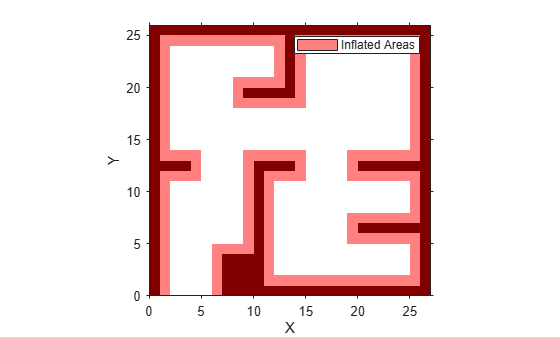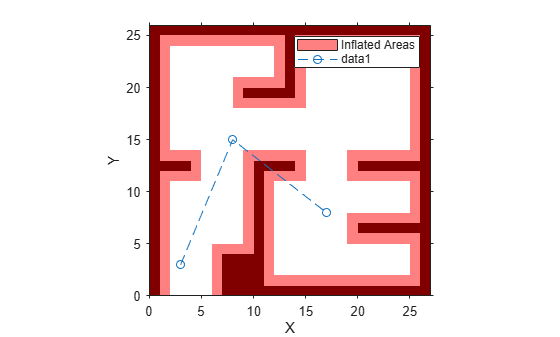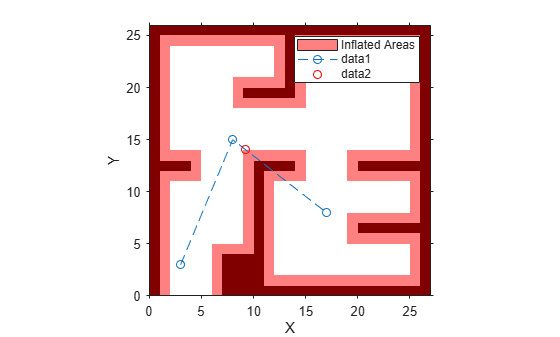validatorVehicleCostmap

State validator based on 2-D costmap

Description

The validatorOccupancyMap object validates states and discretized motions based on the value in a 2-D costmap. An occupied map location is interpreted as an invalid state.

Creation

Description

validator = validatorVehicleCostmap creates a vehicle cost map validator associated with an SE2 state space with default settings.

example

validator = validatorVehicleCostmap(stateSpace) creates a validator in the given state space definition derived from nav.StateSpace.

validator = validatorVehicleCostmap(stateSpace,xyIndices)sets the XYIndices property to specify which variables in the state vector define the xy-coordinates.

validator = validatorVehicleCostmap(stateSpace,Name,Value) specifies the Map or XYIndices properties using Name,Value pair arguments.

Properties

expand all

State space for validating states, specified as a subclass of nav.StateSpace. Provided state space objects include:

Map used for validating states, specified as a vehicleCostMap object.

Interval for sampling between states and checking state validity, specified as a positive numeric scalar.

State variable mapping for xy-coordinates in state vector, specified as a two-element vector, [xIdx yIdx]. For example, if a state vector is given as [r p y x y z], the xy-coordinates are [4 5].

State variable mapping for theta coordinate in state vector, specified as a positive integer. For example, if a state vector is given as [x y theta], the theta coordinate is 3.

Object Functions

 copy Create deep copy of state validator object isStateValid Check if state is valid isMotionValid Check if path between states is valid

Examples

collapse all

This example shows how to validate paths through an evironment.

Load example maps. Use the simple map to create a vehicle cost map. Specify an inflation raidus of 1 meter.

map = vehicleCostmap(double(simpleMap));
plot(map)Specify a coarse path through the map.

path = [3 3 pi/2; 8 15 0; 17 8 -pi/2];
hold on
plot(path(:,1),path(:,2),"--o")Create a state validator using the stateSpaceSE2 definition. Specify the map and the distance for interpolating and validating path segments.

validator = validatorVehicleCostmap(stateSpaceSE2);
validator.Map = map;
validator.ValidationDistance = 0.1;

Check the points of the path are valid states. All three points are in free space, so are considered valid.

isValid = isStateValid(validator,path)
isValid = 3x1 logical array

1
1
1

Check the motion between each sequential path states. The isMotionValid function interpolates along the path between states. If a path segment is invalid, plot the last valid point along the path.

startStates = [path(1,:);path(2,:)];
endStates = [path(2,:);path(3,:)];
for i = 1:2
[isPathValid, lastValid] = isMotionValid(validator,startStates(i,:),endStates(i,:));
if ~isPathValid
plot(lastValid(1),lastValid(2),'or')
end
end
hold off